# Secondary school

1/2 of the pupils want to study at the secondary school, 1/4 at the apprentice, 1/6 at the grammar school 3 pupils do not want to study. How many students are in the class?

Correct result:

n =  36

#### Solution:

n/2+n/4+n/6+3=n

n = 36

n = 36

Calculated by our simple equation calculator.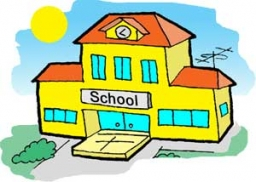We would be very happy if you find an error in the example, spelling mistakes, or inaccuracies, and please send it to us. We thank you!Tips to related online calculators
Need help calculate sum, simplify or multiply fractions? Try our fraction calculator.
Do you have a linear equation or system of equations and looking for its solution? Or do you have quadratic equation?

## Next similar math problems: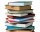Readers borrowed a total of 220 books in the library during the first three days. On the second day, readers borrowed half as many books as the first day and at the same time 20 fewer books than the third day. Depending on the quantity x, express the numb
• Three monksThree medieval monks has task to copy 600 pages of the Bible. One rewrites in three days 1 page, second in 2 days 3 pages and a third in 4 days 2 sides. Calculate for how many days and what day the monks will have copied whole Bible when they begin Wednes
• Sales storesThe first sales store passed the 1/3 and the second 2/5 of the total amount of goods.In third store passed the 2/3 rest of the goods. The remaining 40 kg of goods put into fourth store. How many kilograms of goods pass to a third store?
• Four friendsFour friends shared the money. Vasek got 1/4 of the total amount. Tonda received 1/3 of the rest of the money, Joe got a half from the second residue and Jirka left 80. How much money get together?
• Two numbersThe sum of the two numbers is 1. Find both numbers if you know that half of the first is equal to one seventh of the second number.
• Scratches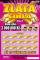The ticket seller sold 1,280 scratch cards in three days. On the second day, he sold 90 tickets less than on the first day, on the third day he sold 1.5 times more tickets than on the second day. How many tickets did he sell on the third day?
• Ice skates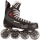Ice skates were raised twice, the first time by 25%, the second time by 10%. After the second price, their cost was 82.5 euros. What was the original price of skates?
• Fifth of the numberThe fifth of the number is by 24 less than that number. What is the number?
• Equation with mixed fractions2 3/5 of 1430+? = 1900. How to do this problem
• Simple equation 6Solve equation with one variable: X/2+X/3+X/4=X+4
• Unknown mixed numberFind the number that is smaller than 5 5/12 by as much as 2 2/13 is smaller than 6 1/6
• Roses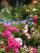On the large rosary was a third white, half red, yellow quarter and six pink. How many roses was in the rosary?
• Balls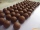From the bag with numbered balls (numbers 1,2,3,. ..20) we pick one ball. What is the probability of choosing a number containing 1?
• Value 4If 5/18=425 what is the value 13/18?
• PC disksPeter has 45 disks in three colors. One-fifth of the disks are blue, red are twice more than the white. How much is blue, red and white disks?
• Reducing numberReducing the an unknown number by 28.5% we get number 243.1. Determine unknown number.
• Cllub flag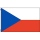The children had the club flag sewn on. The red color covered 2/20 of the total area of the flag, the blue area a fifth, the yellow 19% of the total area. What% is the green area occupied?